# 迭代器和生成器

## 迭代和可迭代

print(dir([1,2]))
print(dir((2,3)))
print(dir({1:2}))
print(dir({1,2}))['__add__', '__class__', '__contains__', '__delattr__', '__delitem__', '__dir__', '__doc__', '__eq__', '__format__', '__ge__', '__getattribute__', '__getitem__', '__gt__', '__hash__', '__iadd__', '__imul__', '__init__', '__iter__', '__le__', '__len__', '__lt__', '__mul__', '__ne__', '__new__', '__reduce__', '__reduce_ex__', '__repr__', '__reversed__', '__rmul__', '__setattr__', '__setitem__', '__sizeof__', '__str__', '__subclasshook__', 'append', 'clear', 'copy', 'count', 'extend', 'index', 'insert', 'pop', 'remove', 'reverse', 'sort']
['__add__', '__class__', '__contains__', '__delattr__', '__dir__', '__doc__', '__eq__', '__format__', '__ge__', '__getattribute__', '__getitem__', '__getnewargs__', '__gt__', '__hash__', '__init__', '__iter__', '__le__', '__len__', '__lt__', '__mul__', '__ne__', '__new__', '__reduce__', '__reduce_ex__', '__repr__', '__rmul__', '__setattr__', '__sizeof__', '__str__', '__subclasshook__', 'count', 'index']
['__class__', '__contains__', '__delattr__', '__delitem__', '__dir__', '__doc__', '__eq__', '__format__', '__ge__', '__getattribute__', '__getitem__', '__gt__', '__hash__', '__init__', '__iter__', '__le__', '__len__', '__lt__', '__ne__', '__new__', '__reduce__', '__reduce_ex__', '__repr__', '__setattr__', '__setitem__', '__sizeof__', '__str__', '__subclasshook__', 'clear', 'copy', 'fromkeys', 'get', 'items', 'keys', 'pop', 'popitem', 'setdefault', 'update', 'values']
['__and__', '__class__', '__contains__', '__delattr__', '__dir__', '__doc__', '__eq__', '__format__', '__ge__', '__getattribute__', '__gt__', '__hash__', '__iand__', '__init__', '__ior__', '__isub__', '__iter__', '__ixor__', '__le__', '__len__', '__lt__', '__ne__', '__new__', '__or__', '__rand__', '__reduce__', '__reduce_ex__', '__repr__', '__ror__', '__rsub__', '__rxor__', '__setattr__', '__sizeof__', '__str__', '__sub__', '__subclasshook__', '__xor__', 'add', 'clear', 'copy', 'difference', 'difference_update', 'discard', 'intersection', 'intersection_update', 'isdisjoint', 'issubset', 'issuperset', 'pop', 'remove', 'symmetric_difference', 'symmetric_difference_update', 'union', 'update']from collections import Iterable

l = [1,2,3,4]
t = (1,2,3,4)
d = {1:2,3:4}
s = {1,2,3,4}

print(isinstance(l,Iterable))
print('__iter__' in dir([l]))
print(isinstance(t,Iterable))
print('__iter__' in dir([t]))
print(isinstance(d,Iterable))
print('__iter__' in dir([d]))
print(isinstance(s,Iterable))
print('__iter__' in dir([s]))

print([1,2].__iter__())

<list_iterator object at 0x1024784a8>iterator，这里给我们标出来了，是一个计算机中的专属名词，叫做迭代器。

# 迭代器协议

'''
dir([1,2].__iter__())是列表迭代器中实现的所有方法，dir([1,2])是列表中实现的所有方法,都是以列表的形式返回给我们的，为了看的更清楚，我们分别把他们转换成集合，然后取差集。
'''
#print(dir([1,2].__iter__()))
#print(dir([1,2]))
print(set(dir([1,2].__iter__()))-set(dir([1,2])))

{'__length_hint__', '__next__', '__setstate__'}

iter_l = [1,2,3,4,5,6].__iter__()
#获取迭代器中元素的长度
print(iter_l.__length_hint__())
#根据索引值指定从哪里开始迭代
print('*',iter_l.__setstate__(4))
#一个一个的取值
print('**',iter_l.__next__())
print('***',iter_l.__next__())

l = [1,2,3,4]
l_iter = l.__iter__()
item = l_iter.__next__()
print(item)
item = l_iter.__next__()
print(item)
item = l_iter.__next__()
print(item)
item = l_iter.__next__()
print(item)
item = l_iter.__next__()
print(item)

l = [1,2,3,4]
l_iter = l.__iter__()
while True:
try:
item = l_iter.__next__()
print(item)
except StopIteration:
breakprint('__next__' in dir(range(12)))  #查看'__next__'是不是在range()方法执行之后内部是否有__next__
print('__iter__' in dir(range(12)))  #查看'__next__'是不是在range()方法执行之后内部是否有__next__

from collections import Iterator
print(isinstance(range(100000000),Iterator))  #验证range执行之后得到的结果不是一个迭代器
range函数的返回值是一个可迭代对象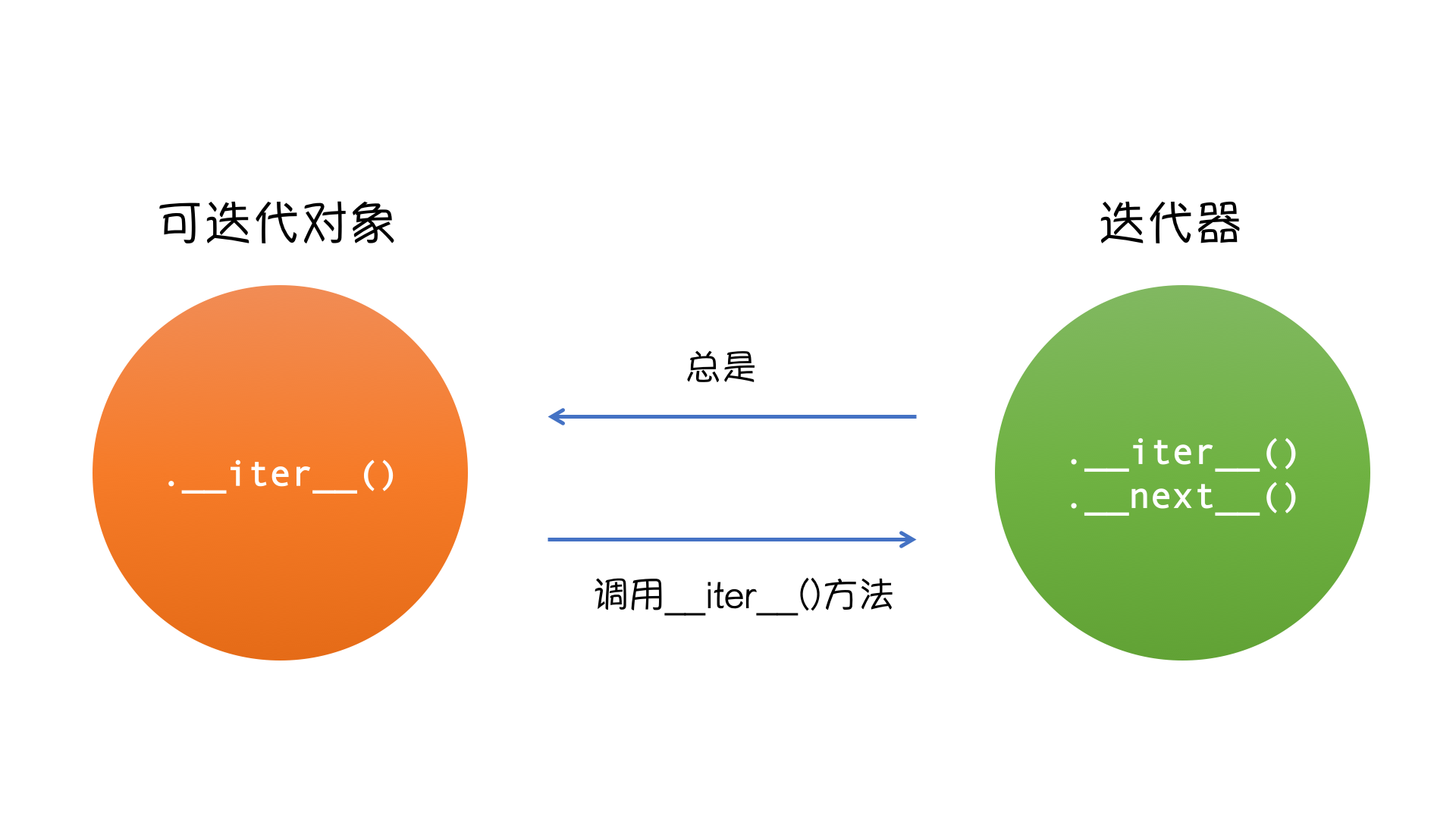### 为什么要有for循环

l=[1,2,3]

index=0
while index < len(l):
print(l[index])
index+=1

#要毛线for循环，要毛线可迭代，要毛线迭代器

## 生成器

### 初识生成器

Python 中提供的 生成器：

1.生成器函数：常规函数定义，但是，使用yield语句而不是return语句返回结果。yield语句一次返回一个结果，在每个结果中间，挂起函数的状态，以便下次从它离开的地方继续执行

2.生成器表达式：类似于列表推导，但是，生成器返回按需产生结果的一个对象，而不是一次构建一个结果列表

### 生成器函数

yield和return一样可以从函数中返回值，但是yield又不同于return，return的执行意味着程序的结束，只能返回一次，yield可以返回多次。

def my_range():
print('我是一个生成器函数')
n = 0
while 1:
yield n
n += 1

def my_range2(start, stop):
n = start
while n < stop:
yield n
n += 1

def my_range3(start, stop, step):
n = start
while n < stop:
yield n
n += step

for i in my_range3(1, 10, 2):
print(i)

#### 更多应用import time

def tail(filename):
f = open(filename)
f.seek(0, 2) #从文件末尾算起
while True:
if not line:
time.sleep(0.1)
continue
yield line

tail_g = tail('tmp')
for line in tail_g:
print(line)

#### send

yield可以返回值，也可以接收值。

def eat(name):
print('%s要开始吃了！' % name)
while 1:
food = yield
print('{}在吃{}'.format(name, food))

a = eat('alex')
a.__next__()  # 初始化，让函数暂停在yield处
a.send('包子')  # send两个作用：1.给yield传值 2.继续执行函数
a.send('饺子')

yield可以同时返回值和接收值。def averager():
total = 0.0
count = 0
average = None
while True:
term = yield average
total += term
count += 1
average = total/count

g_avg = averager()
next(g_avg)
print(g_avg.send(10))
print(g_avg.send(30))
print(g_avg.send(5))def init(func):  #在调用被装饰生成器函数的时候首先用next激活生成器
def inner(*args,**kwargs):
g = func(*args,**kwargs)
next(g)
return g
return inner

@init
def averager():
total = 0.0
count = 0
average = None
while True:
term = yield average
total += term
count += 1
average = total/count

g_avg = averager()
# next(g_avg)   在装饰器中执行了next方法
print(g_avg.send(10))
print(g_avg.send(30))
print(g_avg.send(5))

#### yield fromdef gen1():
for c in 'AB':
yield c
for i in range(3):
yield i

print(list(gen1()))

def gen2():
yield from 'AB'
yield from range(3)

print(list(gen2()))
yield from

## 列表推导式和生成器表达式#老男孩由于峰哥的强势加盟很快走上了上市之路,alex思来想去决定下几个鸡蛋来报答峰哥

egg_list=['鸡蛋%s' %i for i in range(10)] #列表解析

#峰哥瞅着alex下的一筐鸡蛋,捂住了鼻子,说了句:哥,你还是给我只母鸡吧,我自己回家下

laomuji=('鸡蛋%s' %i for i in range(10))#生成器表达式
print(laomuji)
print(next(laomuji)) #next本质就是调用__next__
print(laomuji.__next__())
print(next(laomuji))

1.把列表解析的[]换成()得到的就是生成器表达式

2.列表解析与生成器表达式都是一种便利的编程方式，只不过生成器表达式更节省内存

3.Python不但使用迭代器协议，让for循环变得更加通用。大部分内置函数，也是使用迭代器协议访问对象的。例如， sum函数是Python的内置函数，该函数使用迭代器协议访问对象，而生成器实现了迭代器协议，所以，我们可以直接这样计算一系列值的和：

sum(x ** 2 for x in range(4))

sum([x ** 2 for x in range(4)])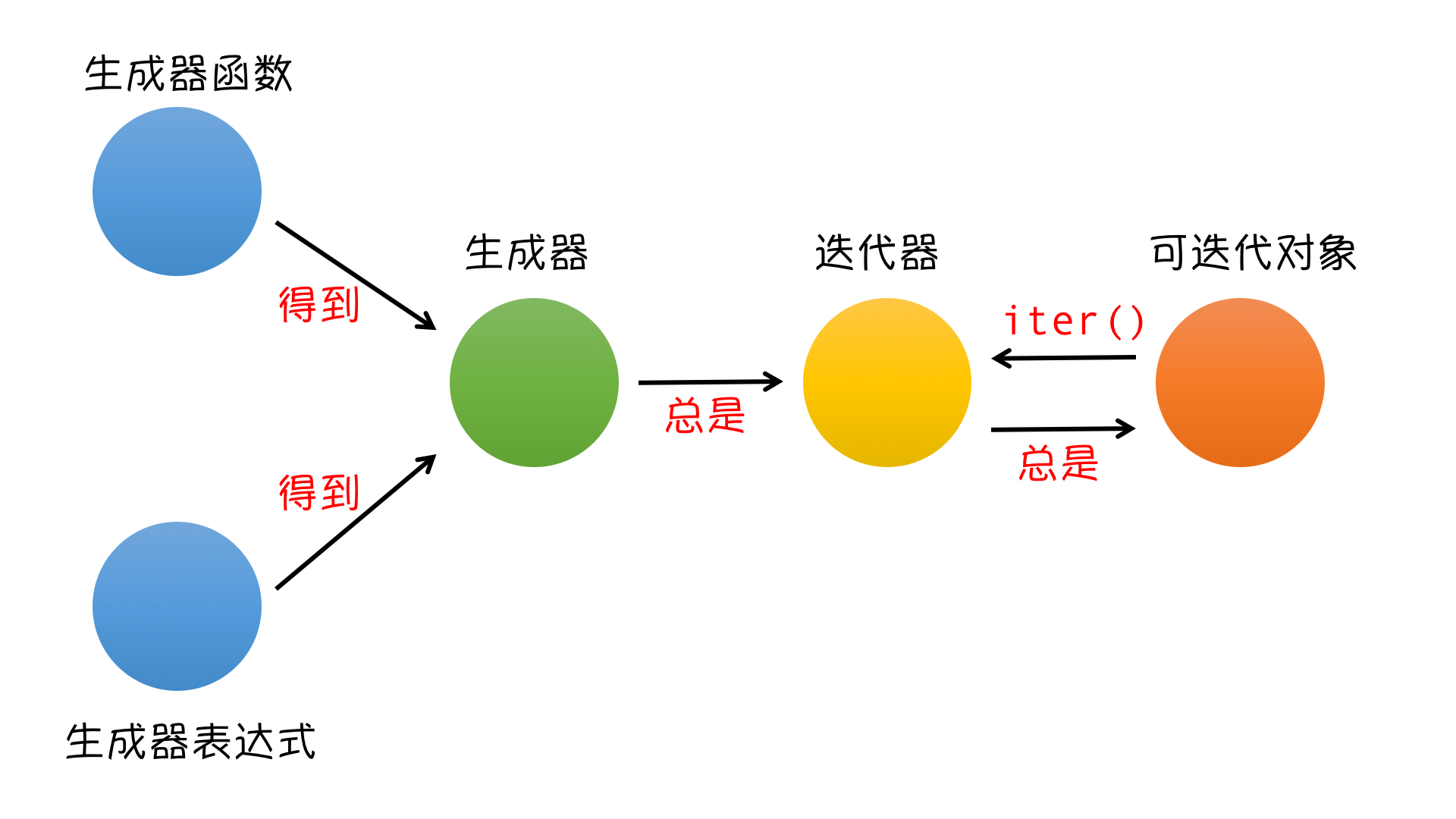更多精彩请见——迭代器生成器专题：https://www.cnblogs.com/liwenzhou/p/9762052.html

## 生成器相关的面试题def demo():
for i in range(4):
yield i

g=demo()

g1=(i for i in g)
g2=(i for i in g1)

print(list(g1))
print(list(g2))def add(a, b):
return a + b

def func():
for i in range(4):
yield i

g = func()
for n in [1, 10]:
g = (add(n, i) for i in g)

print(list(g))

# 内置函数

Built-in Functions
abs() dict() help() min() setattr()
all() dir() hex() next() slice()
any() divmod() id() object() sorted()
ascii() enumerate() input() oct() staticmethod()
bin() eval() int() open() str()
bool() exec() isinstance() ord() sum()
bytearray() filter() issubclass() pow() super()
bytes() float() iter() print() tuple()
callable() format() len() property() type()
chr() frozenset() list() range() vars()
classmethod() getattr() locals() repr() zip()
compile() globals() map() reversed() __import__()
complex() hasattr() max() round()
delattr() hash() memoryview() set()

## abs/round/sum

>>> abs(1)
1
>>> abs(-1)         # 求绝对值
1
>>> round(1.234,2)
1.23
>>> round(1.269,2)  # 四舍五入
1.27
>>> sum([1,2,3,4])
10
>>> sum((1,3,5,7))  # 接收数字组成的元组/列表
16

## callable/chr/dir

>>> def func():pass  >>> callable(func)   # 判断一个变量是否可以调用 函数可以被调用
True
>>> a = 123          # 数字类型a不能被调用
>>> callable(a)
False
>>> chr(97)          # 将一个数字转换成一个字母
'a'
>>> chr(65)
'A'
>>> dir(123)         # 查看数字类型中含有哪些方法
['__abs__', '__add__', '__and__', '__bool__', '__ceil__', '__class__', '__delattr__', '__dir__', '__divmod__', '__doc__', '__eq__', '__float__', '__floor__', '__floordiv__', '__format__', '__ge__', '__getattribute__', '__getnewargs__', '__gt__', '__hash__', '__index__', '__init__', '__init_subclass__', '__int__', '__invert__', '__le__', '__lshift__', '__lt__', '__mod__', '__mul__', '__ne__', '__neg__', '__new__', '__or__', '__pos__', '__pow__', '__radd__', '__rand__', '__rdivmod__', '__reduce__', '__reduce_ex__', '__repr__', '__rfloordiv__', '__rlshift__', '__rmod__', '__rmul__', '__ror__', '__round__', '__rpow__', '__rrshift__', '__rshift__', '__rsub__', '__rtruediv__', '__rxor__', '__setattr__', '__sizeof__', '__str__', '__sub__', '__subclasshook__', '__truediv__', '__trunc__', '__xor__', 'bit_length', 'conjugate', 'denominator', 'from_bytes', 'imag', 'numerator', 'real', 'to_bytes']
>>> dir('abc')
['__add__', '__class__', '__contains__', '__delattr__', '__dir__', '__doc__', '__eq__', '__format__', '__ge__', '__getattribute__', '__getitem__', '__getnewargs__', '__gt__', '__hash__', '__init__', '__init_subclass__', '__iter__', '__le__', '__len__', '__lt__', '__mod__', '__mul__', '__ne__', '__new__', '__reduce__', '__reduce_ex__', '__repr__', '__rmod__', '__rmul__', '__setattr__', '__sizeof__', '__str__', '__subclasshook__', 'capitalize', 'casefold', 'center', 'count', 'encode', 'endswith', 'expandtabs', 'find', 'format', 'format_map', 'index', 'isalnum', 'isalpha', 'isascii', 'isdecimal', 'isdigit', 'isidentifier', 'islower', 'isnumeric', 'isprintable', 'isspace', 'istitle', 'isupper', 'join', 'ljust', 'lower', 'lstrip', 'maketrans', 'partition', 'replace', 'rfind', 'rindex', 'rjust', 'rpartition', 'rsplit', 'rstrip', 'split', 'splitlines', 'startswith', 'strip', 'swapcase', 'title', 'translate', 'upper', 'zfill']

## eval/exec

>>> eval('1+2-3*4/5')  # 执行字符串数据类型的代码并且将值返回
0.6000000000000001
>>> exec('print(123)') # 执行字符串数据类型的代码但没有返回值
123

## enumerate

>>> enumerate(['apple','banana'],1)   # 会给列表中的每一个元素拼接一个序号
<enumerate object at 0x113753fc0>
>>> list(enumerate(['apple','banana'],1))
[(1, 'apple'), (2, 'banana')]

## max/min

>>> max(1,2,3,)     # 求最小值
3
>>> min(2,1,3)      # 求最大值
1

## sorted

>>> sorted([1, 4, 5, 12, 45, 67])  # 排序，并生成一个新的有序列表
[1, 4, 5, 12, 45, 67]

>>> sorted([1, 4, 5, 12, 45, 67], reverse=True)
[67, 45, 12, 5, 4, 1]
list1 = [
{'name': 'Zoe', 'age': 30},
{'name': 'Bob', 'age': 18},
{'name': 'Tom', 'age': 22},
{'name': 'Jack', 'age': 40},
]

ret = sorted(list1, key=lambda x: x['age'])
print(ret)

# [{'name': 'Bob', 'age': 18}, {'name': 'Tom', 'age': 22}, {'name': 'Zoe', 'age': 30}, {'name': 'Jack', 'age': 40}]

## zip

zip函数接收一个或多个可迭代对象作为参数，最后返回一个迭代器：

>>> x = ["a", "b", "c"]
>>> y = [1, 2, 3]
>>> a = list(zip(x, y))  # 合包
>>> a
[('a', 1), ('b', 2), ('c', 3)]
>>> b =list(zip(*a))  # 解包
>>> b
[('a', 'b', 'c'), (1, 2, 3)]

zip(x, y) 会生成一个可返回元组 (m, n) 的迭代器，其中m来自x，n来自y。 一旦其中某个序列迭代结束，迭代就宣告结束。 因此迭代长度跟参数中最短的那个序列长度一致。

>>> x = [1, 3, 5, 7, 9]
>>> y = [2, 4, 6, 8]
>>> for m, n in zip(x, y):
...   print(m, n)
...
1 2
3 4
5 6
7 8

>>> from itertools import zip_longest
>>> x = [1, 3, 5, 7, 9]
>>> y = [2, 4, 6, 8]
>>> for m, n in zip_longest(x, y):
...   print(m, n)
...
1 2
3 4
5 6
7 8
9 None

zip其他常见应用：

>>> keys = ["name", "age", "salary"]
>>> values = ["Andy", 18, 50]
>>> d = dict(zip(keys, values))
>>> d
{'name': 'Andy', 'age': 18, 'salary': 50}

## map

map()接收两个参数func（函数）和seq（序列，例如list）。如下图：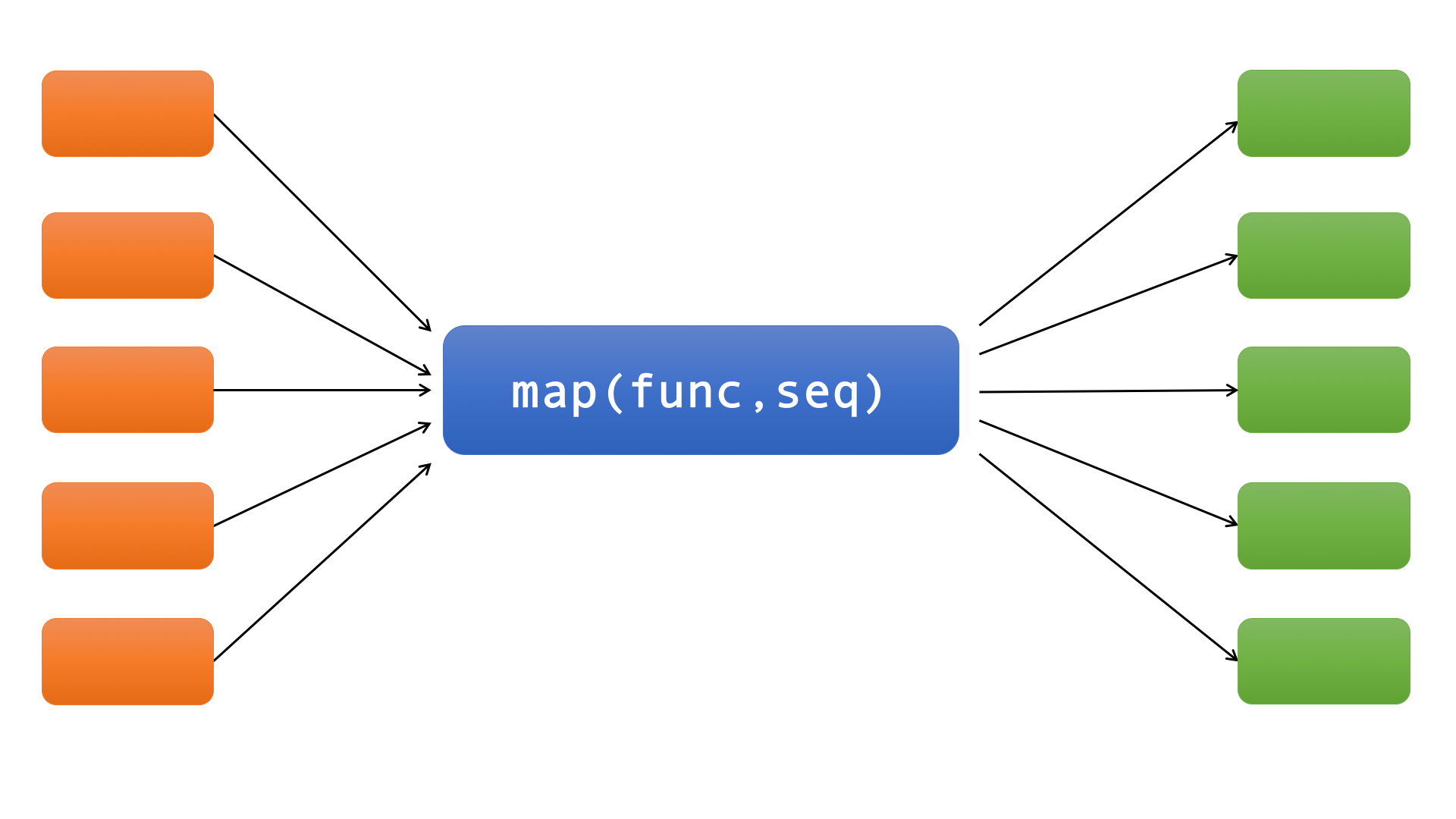map()将函数func应用于序列seq中的所有元素。在Python3之前，map()返回一个列表，列表中的每个元素都是将列表或元组“seq”中的相应元素传入函数func返回的结果。Python 3中map()返回一个迭代器。

>>> l = [11, 22, 33, 44, 55]
>>> list(map(lambda x:x+100, l))
[111, 122, 133, 144, 155]

def my_map(func, seq):
result = []
for i in seq:
result.append(func(i))
return result

>>> def my_map(func, seq):
...     result = []
...     for i in seq:
...         result.append(func(i))
...     return result
...
>>> l = [11, 22, 33, 44, 55]
>>> list(my_map(lambda x:x+100, l))
[111, 122, 133, 144, 155]

def my_map(func, seq):
for i in seq:
yield func(i)

>>> def my_map(func, seq):
...     for i in seq:
...         yield func(i)
...
>>> l = [11, 22, 33, 44, 55]
>>> list(my_map(lambda x:x+100, l))
[111, 122, 133, 144, 155]

>>> pow(2, 10)
1024
>>> pow(3, 11)
177147
>>> pow(4, 12)
16777216
>>> list(map(pow, [2, 3, 4], [10, 11, 12]))
[1024, 177147, 16777216]

## filter

filter函数和map函数一样也是接收两个参数func（函数）和seq(序列，如list)，如下图：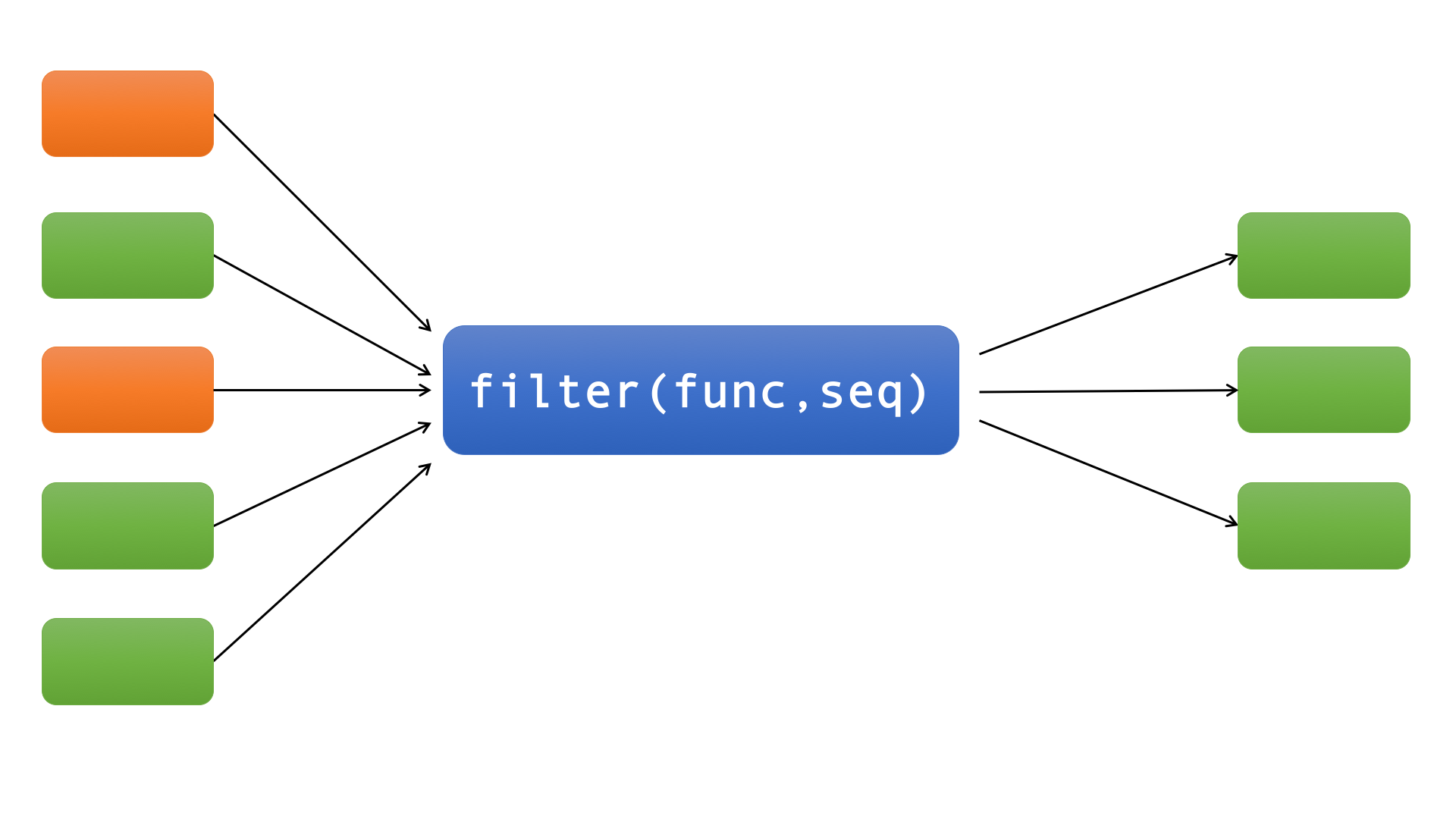filter函数类似实现了一个过滤功能，它过滤序列中的所有元素，返回那些传入func后返回True的元素。也就是说filter函数的第一个参数func必须返回一个布尔值，即True或者False。

>>> l = [30, 11, 77, 8, 25, 65, 4]
>>> list(filter(lambda x: x>33, l))
[77, 65]

>>> x = [1, 2, 3, 5, 6]
>>> y = [2, 3, 4, 6, 7]
>>> list(filter(lambda a: a in y, x))
[2, 3, 6]

### 补充：reduce

reduce

reduce同样是接收两个参数：func（函数）和seq(序列，如list)，如下图：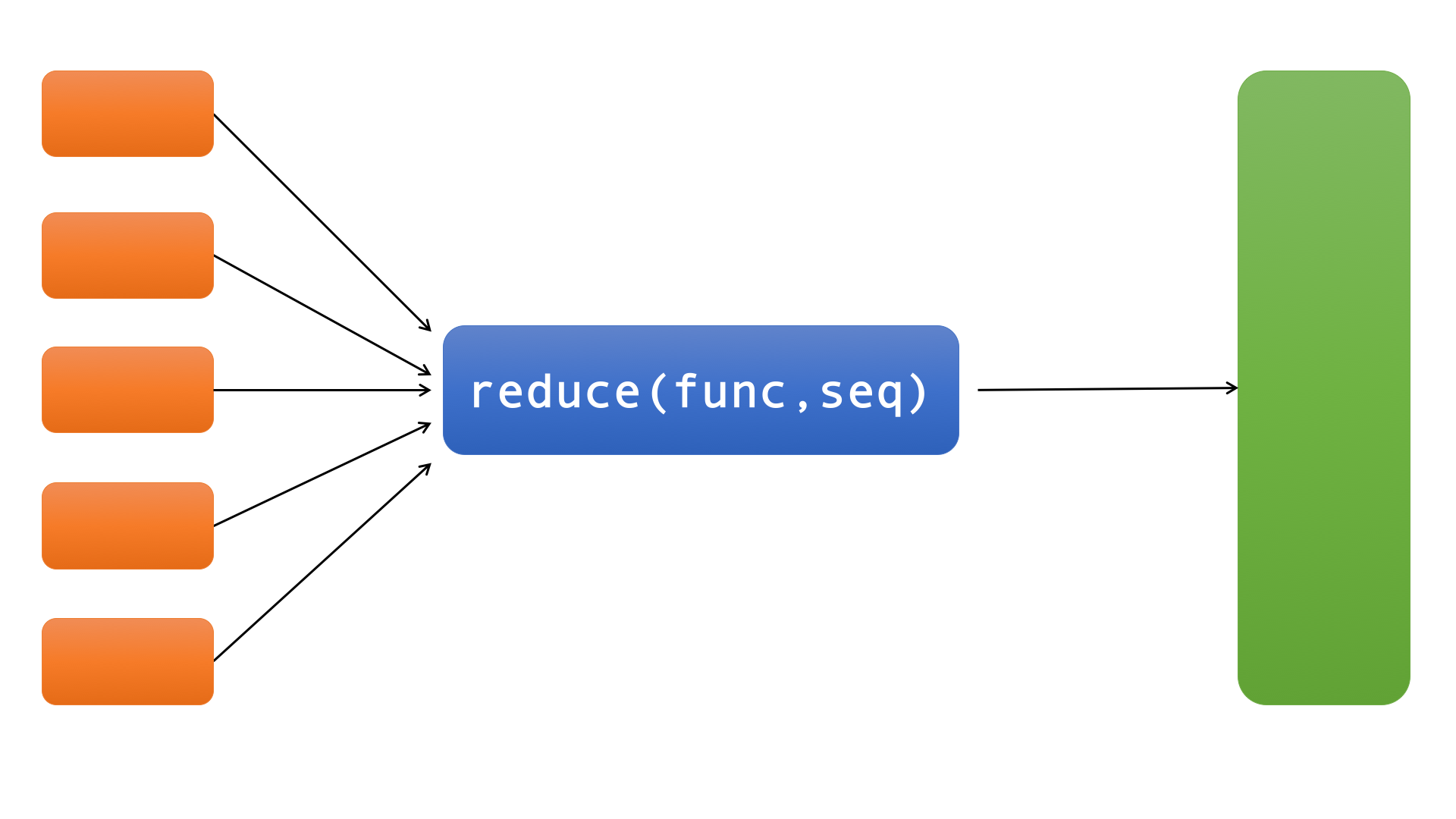reduce最后返回的不是一个迭代器，它返回一个值。

reduce首先将序列中的前两个元素，传入func中，再将得到的结果和第三个元素一起传入func，…，这样一直计算到最后，得到一个值，把它作为reduce的结果返回。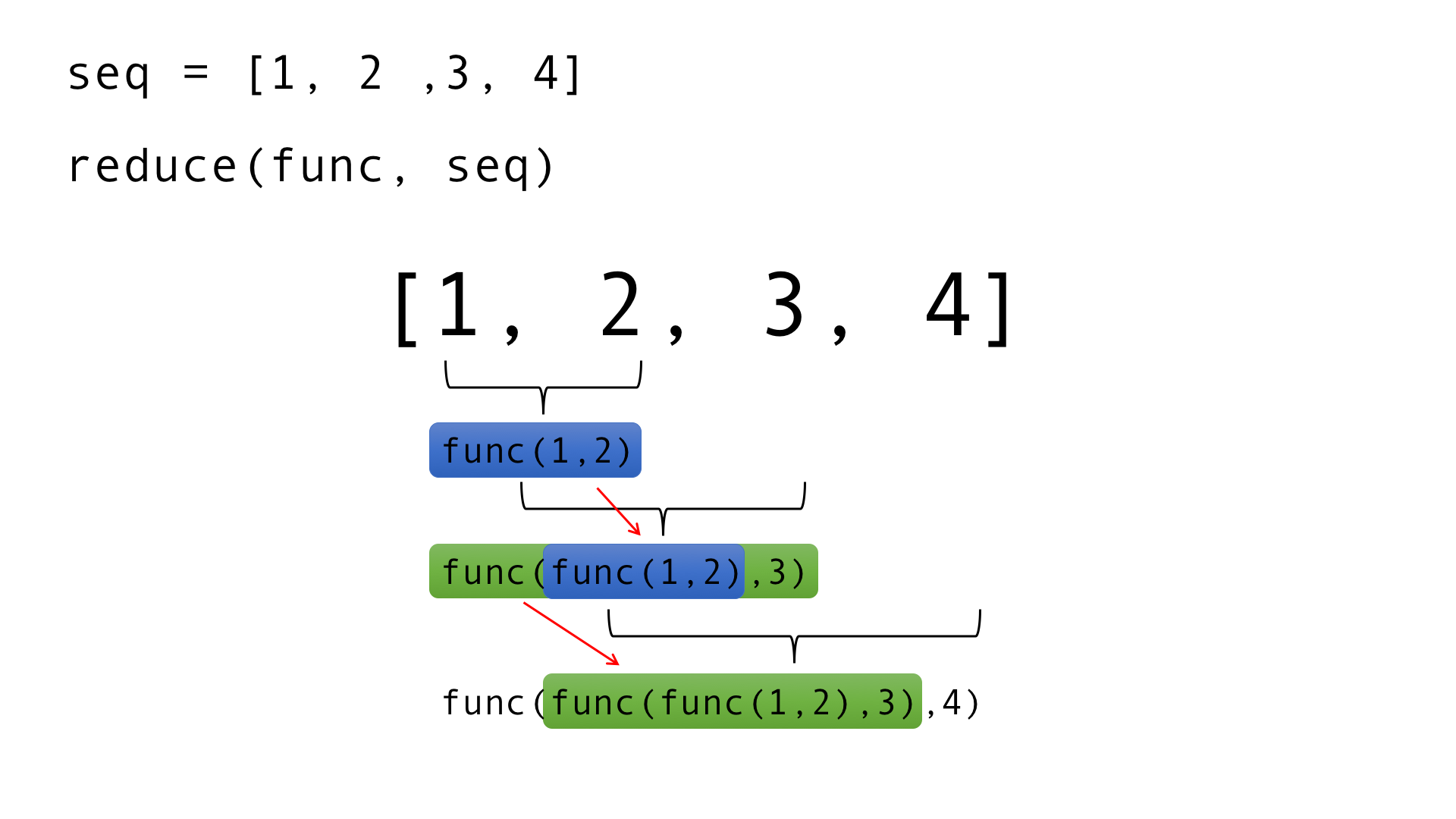>>> from functools import reduce
>>> reduce(lambda x,y:x+y, [1, 2, 3, 4])
10

>>> from functools import reduce
>>> reduce(lambda x,y:x+y, range(1, 101))
5050

lambda

lambda是匿名函数，也就是没有名字的函数。lambda的语法非常简单：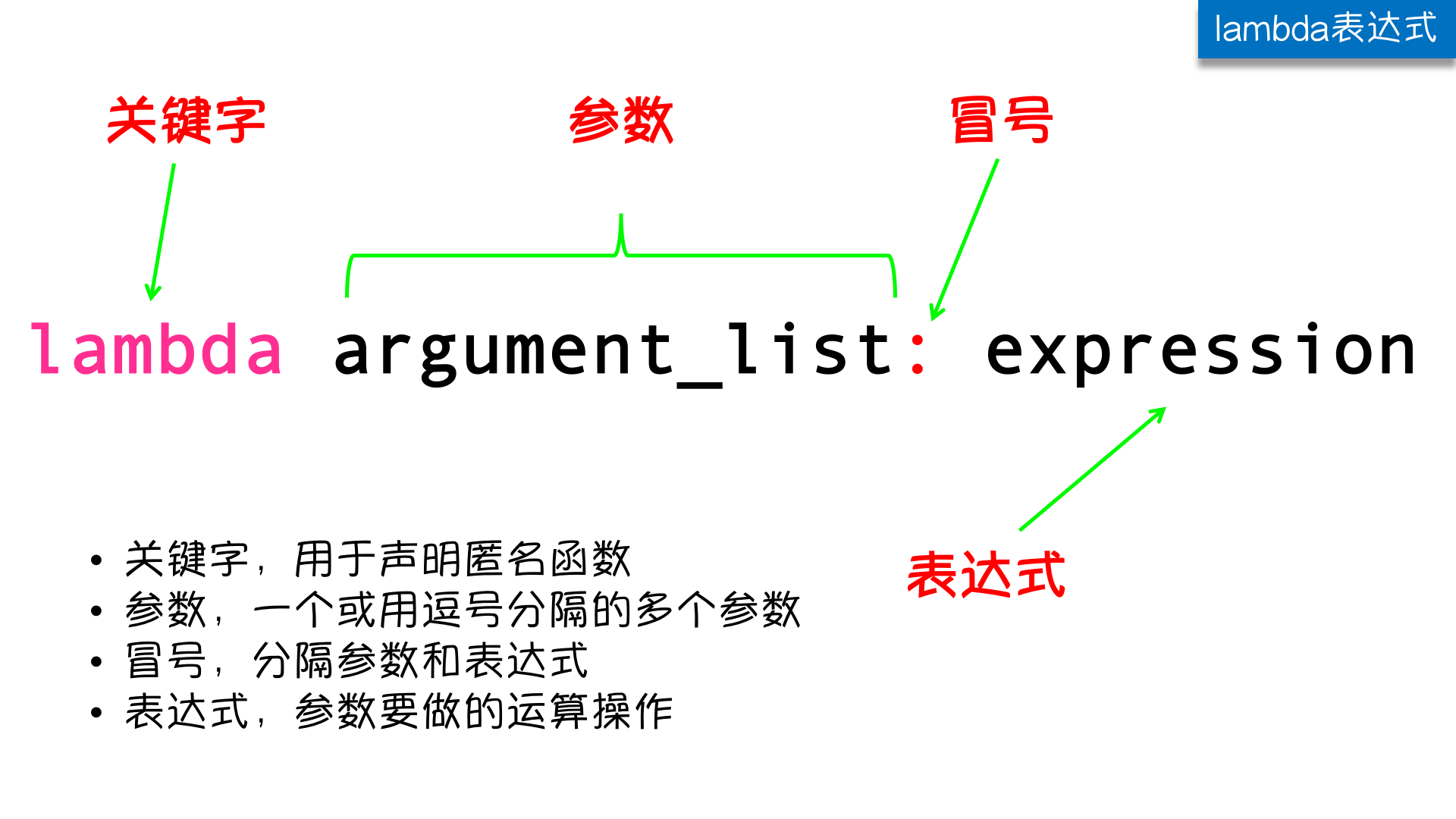#这段代码
def func(x, y):
return x + y#换成匿名函数
lambda x, y:x+y

lambda表达式和定义一个普通函数的对比：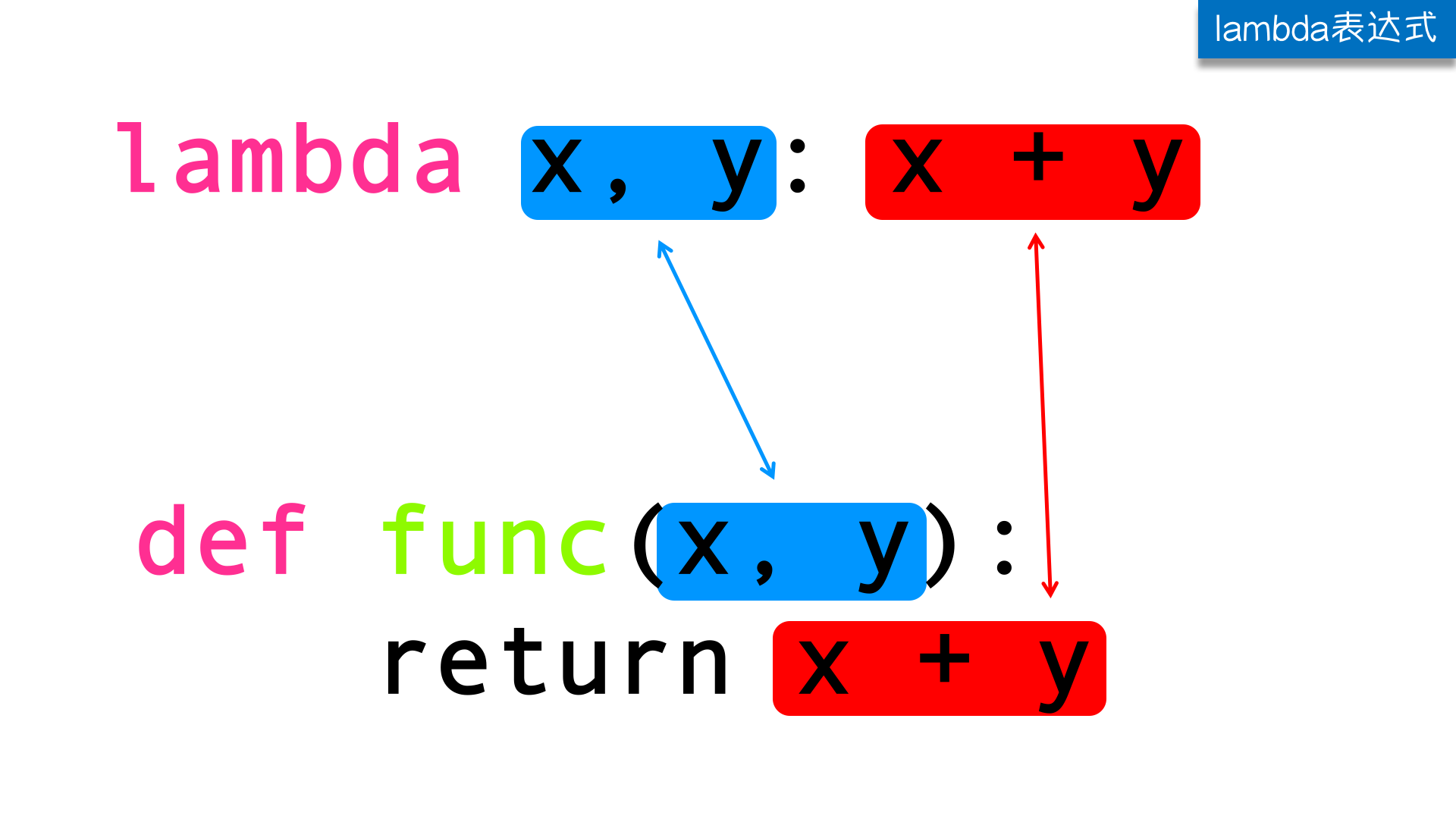请把以下函数变成匿名函数
def func(x, y):
return x + y

l=[3,2,100,999,213,1111,31121,333]
print(max(l))

dic={'k1':10,'k2':100,'k3':30}

print(max(dic))
print(dic[max(dic,key=lambda k:dic[k])])
res = map(lambda x:x**2,[1,5,7,4,8])
for i in res:
print(i)

1
25
49
16
64
res = filter(lambda x:x>10,[5,8,11,9,15])
for i in res:
print(i)

11
15

1.现有两个元组(('a'),('b')),(('c'),('d'))，请使用python中匿名函数生成列表[{'a':'c'},{'b':'d'}]#答案一
test = lambda t1,t2 :[{i:j} for i,j in zip(t1,t2)]
print(test(t1,t2))
#答案二
print(list(map(lambda t:{t:t},zip(t1,t2))))
#还可以这样写
print([{i:j} for i,j in zip(t1,t2)])1.下面程序的输出结果是：
d = lambda p:p*2
t = lambda p:p*3
x = 2
x = d(x)
x = t(x)
x = d(x)
print x



# 三元运算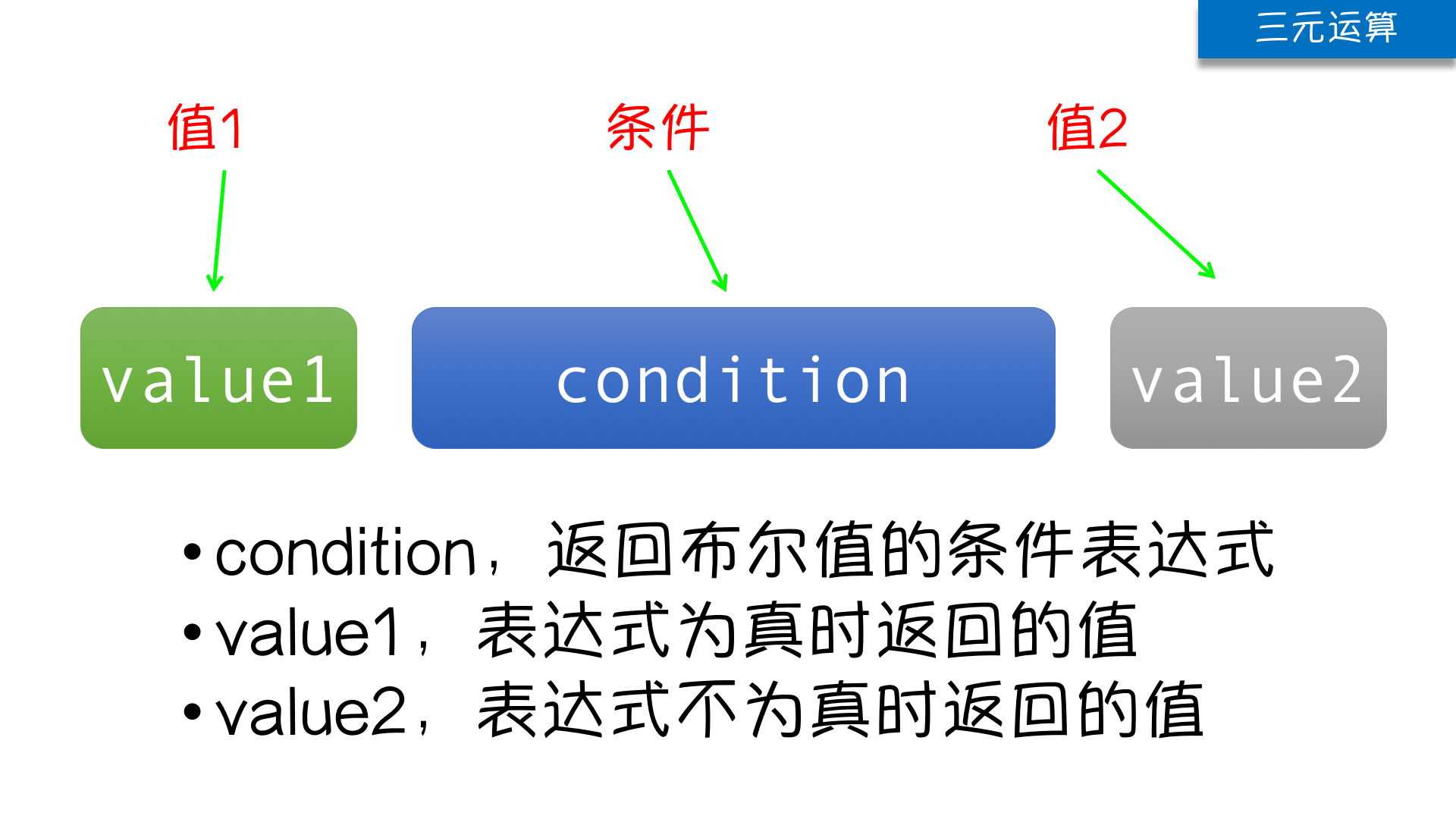>>> from functools import reduce
>>> l = [30, 11, 77, 8, 25, 65, 4]
>>> reduce(lambda x,y: x if x > y else y, l)
77

>>> l = [30, 11, 77, 8, 25, 65, 4]
>>> list(map(lambda x: x+100 if x%2 else x, l))
[30, 111, 177, 8, 125, 165, 4]

# 递归

def story():
s = """
从前有个山，山里有座庙，庙里有个老和尚在讲故事，
讲的什么呢？
"""
print(s)
story()

story()

### 初识递归

Python为了杜绝此类现象，强制的将递归层数控制在了1000 (你写代码测试可能只测出997或998)。

import sys
print(sys.getrecursionlimit())

1000是Python为了我们程序的内存优化所设定的一个默认值，我们当然还可以通过一些手段去修改它：import sys
print(sys.setrecursionlimit(100000))

### 再谈递归

1. 子问题须与原始问题为同样的事，且更为简单（问题相同，但规模在变小）；

2. 不能无限制地调用本身，须有个出口，化简为非递归状况处理。

def tell(x):
for i in x:
if not isinstance(i, list):
print(i)
else:
tell(i)

tell(list1)

### 递归函数与二分查找算法

https://www.cnblogs.com/liwenzhou/p/9762074.html

posted @ 2018-10-09 16:22  Q1mi  阅读(2889)  评论(0编辑  收藏  举报